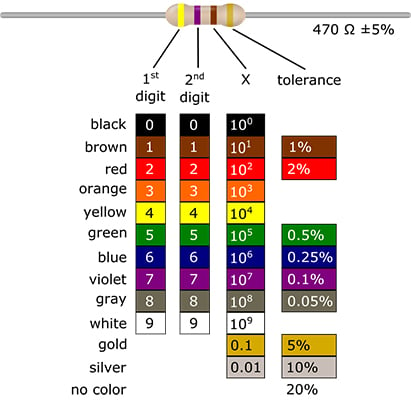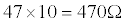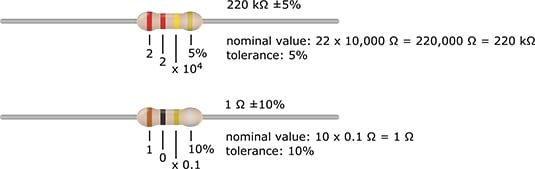##### Electronics For Kids For DummiesIf you think those colorful bands on your resistors are there just for show, think again! Those bands tell you the value of the resistor. Before you can decode the resistor value, you need to know a little more about resistors.

There are two main types of resistors:

• Standard resistors have four color bands. Three of the bands tell you the nominal value, which means the value the resistor was designed to have. The fourth band tells you the tolerance of the resistor, which indicates how far off the nominal value the actual resistance could be. (The manufacturing process isn't perfect, so most resistors are a little off.)

For instance, you may buy what you think is a 100 Omega resistor, but the actual resistance most likely isn't exactly 100 Omega. It may be 97 or 104 Omega, or some other value close to 100 Omega. For most circuits, "close" is good enough.

• Precision resistors, which have more precise values than standard resistors, have five color bands. Four of the bands tell you the nominal value. The fifth band tells you the tolerance.

You can count on the actual resistance of a precision resistor being really close to its nominal value. So, if you buy a 100 Omega precision resistor, chances are its actual value is within 1 or 2 of 100 Omega.

The following figure shows a diagram of the color code of a standard (four-band) resistor. You use this color code to figure out the nominal value and tolerance of a standard resistor.## Decoding the nominal value of a resistor

Here's how you use the color code to figure out the nominal value of the resistor (refer to the figure):

1. Decide which band is the first band.

Compare the ends of the resistor. Usually, the colored band at one end is closer to that end than is the colored band at the other end. If that is the case, the band that is closest to one end of the resistor is the first band.

If you can't determine which is the first band, look at the two outer bands. If one of the outer bands is silver or gold, that band is probably the last band, so the first band is at the other end.

2. Look up the color of the first band in the column labeled "1st digit" and find the number associated with that color.

This number is the first digit of the resistance. In the resistor shown in the preceding figure, the first band is yellow, so the first digit is 4.

3. Look up the color of the second band in the column labeled "2nd digit" and find the number associated with that color.

This number is the second digit of the resistance. In the resistor shown in the preceding figure, the second band is violet, so the second digit is 7.

4. Look up the color of the third band in the column labeled "X" and find the number associated with that color.

This number is the multiplier. In the resistor shown in the preceding figure, the third band is brown, so the multiplier is 101 (which is 10).

5. Put the first two digits side-by-side to form a two-digit number.

For the resistor shown in the preceding figure, the first two digits are 4 and 7, so the two-digit number is 47.

6. Multiply the two-digit number by the multiplier.

This gives you the nominal value of the resistor in ohms. In the resistor shown in the preceding figure, the two-digit number is 47 and the multiplier is 10, so the nominal value isAn easy way to multiply a whole number by a power of 10 (that is, 100, 101, 102, 103, and so on) is to just append (meaning tack on to the end) the whole number with zeros, and use the exponent (which is the small, raised number next to the 10) to tell you how many zeros to append. Here are two examples:

• 22 x 103. The exponent is 3, so you stick 3 zeros to the right of 22, and you get 22,000. (The multiplier in this case is 103, which is 1,000.)

• 56 x 100. The exponent is 0, so you stick 0 zeros to the right of 56, and you get 37. (The multiplier in this case is 100, which is 1, because any number raised to the 0th power equals 1.)

If you have a precision (five-band) resistor (which you're unlikely to use for projects in Electronics For Kids For Dummies), the third band gives you the third digit of the resistance and the fourth band gives you the multiplier.

To figure out how far off from the nominal value the actual resistance could be, you look at the fourth band on a standard resistor (or the fifth band on a precision resistor). Refer to the preceding figure for the color code for the tolerance of a resistor.

Say that the fourth band of the 470 Omega resistor you have chosen for a particular project is gold. The color, gold, in the column labeled "tolerance" in the figure represents a tolerance of 5 percent. Because 5 percent of 470 is 23.5, the actual resistance could be as much as 23.5 Omega higher or lower than 470 Omega. So the actual value of the resistance could be any value from 446.5 to 493.5 Ω.

Most standard resistors have tolerances of 5%, 10%, or 20%, and most precision resistors have tolerances of 1% or 2%. For the majority of circuits — and in all the projects in Electronics For Kids For Dummies — it's okay to use a standard resistor. For certain circuits, it's important to use a precision resistor with a lower tolerance.

The following figure shows you two more examples of resistors and their values.You can measure the actual value of a specific resistor using a device called a multimeter. For example, when you use a multimeter to measure a 470 Omega resistor with a 5 percent tolerance, you might found that the actual value is 481 Omega.